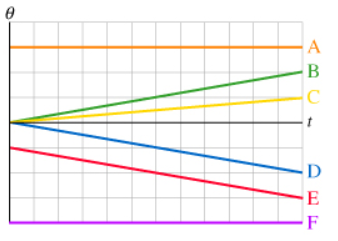# Problem: The figure shows angular position versus time graphs for six different objects.Rank these graphs on the basis of the angular acceleration of the object. Rank positive angular accelerations as larger than negative angular accelerations.Rank from largest to smallest. To rank items as equivalent, overlap them.

###### FREE Expert Solution

For A and F, the position is not changing. Thus, the objects are stationary. Both the velocity and angular acceleration is zero.

94% (56 ratings)###### Problem Details

The figure shows angular position versus time graphs for six different objects.Rank these graphs on the basis of the angular acceleration of the object. Rank positive angular accelerations as larger than negative angular accelerations.

Rank from largest to smallest. To rank items as equivalent, overlap them.